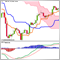• 概述
• 评论 (1)
• 评论 (2)
• 新特性

# Brilliant Keltner Channel MT4

This indicator draws the Keltner Channel for any time frame Displayed on the current Time frame allowing user to choose which moving average type for the calculations.

The available Moving Averages are:

• Simple Moving Average (MA_Mode=Mode_SMA)
• Exponential Moving Average (MA_Mode=Mode_EMA)
• Smoothed Moving Average (MA_Mode=Mode_SmoothedAverage)
• Linear Weighted Moving Average (MA_Mode=Mode_LinearWeighted)
• Tillson's Moving Average (MA_Mode=Mode_Telsons)

Moving Average line is coded into RED or BLUE according to its direction from the previous candle.

User can choose to calculate the bands of the channel according to ATR or using Standard Deviation with confidence value

### Parameters:

• MaxBars: the maximum number of candles to calculate for.
• TimeFrame: the desired time frame to calculate for.
• MA_Period: Keltner channel Moving average Period for calculations.
• MA_Mode: Moving Avergae Modes
• Simple Moving Average (MA_Mode=Mode_SMA)
• Exponential Moving Average (MA_Mode=Mode_EMA)
• Smoothed Moving Average (MA_Mode=Mode_SmoothedAverage)
• Linear Weighted Moving Average (MA_Mode=Mode_LinearWeighted)
• Tillson's Moving Average (MA_Mode=Mode_Telsons)
• MA_Price: the price to be used for calculations.
• MA_Volume: the volume coefficient to be used when using Tillson's moving average.
• UseATR: True to use ATR for calculating Keltner channel boundaries. False will use standard deviation.
• VarianceCalcBars: number of bars to use in calculating variance for the desired time frame.
• ConfidencePercentage: the percentage to be multiplied with the standard deviation when used.
• ATR_Period: Period used for ATR calculations.
• ATR_Multiply: coefficient to be multiplied with the ATR value.

it can be used with "Brilliant_Advanced_Timing" https://www.mql5.com/en/market/product/31706 for finding a proper Entering Point.2444
2017.03.15 18:04

Works great

This Version Introduces TimeFrame Calculation concept, which allow user to choose to calculate the keltner channel for any higher time frame and show it on the current time frame.
also a more friendly way to specify the moving average price